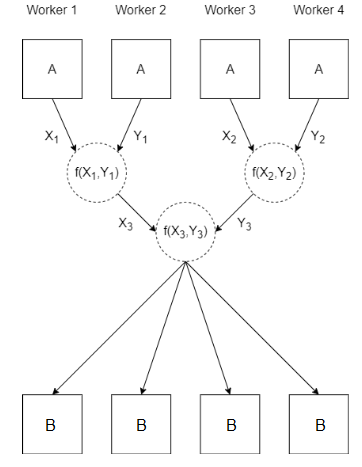# gop

Reduce arrays on `spmd` workers

## Syntax

``B = gop(fcn,A)``
``B = gop(fcn,A,destination)``

## Description

example

````B = gop(fcn,A)` uses the function `fcn` to reduce the array `A` defined on each worker running an `spmd` block or communicating job.For example, `gop(@plus,A)` returns the sum of the arrays `A` defined on each worker.MATLAB uses the `fcn` function to reduce the array `A` defined on each worker by calling the function `N-1` times. `N` is the value returned by `numlabs` in that `spmd` block or communicating job.Aj is the array `A` as defined on `spmd` worker `j`.When you use `parfor`, `parfeval`, or `parfevalOnAll` to run code on a parallel pool, the workers are independent and do not communicate with each other. If you use `gop` on these workers, the result is the same as `gop` on a client.If `numlabs` is equal to `1`, `B` is equal to `A`.```
````B = gop(fcn,A,destination)` reduces the array `A` defined on each worker and stores the result on only one worker.```

## Examples

collapse all

This example shows how to use `gop` to calculate the maximum value for `x` across all workers.

Create a parallel pool with 4 workers.

`parpool(4);`

When you execute an `spmd` block after creating a parallel pool, by default all available workers in the pool will run the code inside the `spmd` block.

Run `labindex` on each worker in the `spmd` block, and store the result in a scalar `A`. Use `gop` and `max` to calculate the maximum value of `A` from each worker.

When you use `gop`, by default the result is stored on every worker. On the client, `C` is a `Composite` array. To get the result, index into the `Composite` array.

```spmd A = labindex; C = gop(@max,A); end disp(C{1})```
` 4`

## Input Arguments

collapse all

Input array, specified as any MATLAB variable.

Example: `magic(3)`

Reduction function, specified as a function handle. The function must take two input arguments.

Example: `fcn = @max`

Data Types: `function_handle`

Index of destination worker, specified as a positive integer scalar. The value must be less than or equal to the value given by `numlabs`, the number of workers running the current `spmd` block or communicating job. When specified, the result of `gop(fcn,A,destination)` is only stored on one worker. On the worker with `labindex` equal to `destination`, `C` is the result of the operation. On all other workers, `C` is `[]`.

Example: `1`

## Algorithms

When you use `gop(fcn,A)`, `fcn` is used to combine the value of `A` from each worker.

To ensure that your `spmd` block or communicating job always produces the same results, specify `fcn` as an associative function.## Version History

Introduced before R2006a